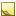﻿[Defaults] section[Defaults] section

Every quantifiable data type used for measurements has a default unit of measure. You can override the default units template-wide in the [Defaults] section, which removes the need to specify your preferred unit of measure within each individual template line.

Once you define your preferred units of measure in [Defaults], every column of that data type in your template defaults to the specified default unit.

For the name/value pairs in this section:

• The name portion (left side) declares the data type for which you want to set your preferred default unit.
• The value portion (right side) declares the default unit of measure.Note

If you list any unsupported data types or units of measure in the [Defaults] section, then an error will be returned when you run the template.

Default units of measure can be set for the following quantifiable data types in the [Defaults] section:

The default values you set apply to any columns of that data type used in the following Template sections:

This topic contains the following sections:Setting a default

In the [Default] section, list each data type and preferred unit of measure, as shown below.

Retrieve template
```[Template]
TemplateVersion = 1.0
Intent = Retrieve
OrderBy = Input.TimeTriggered

[Defaults]
Speed = kph     # Sets kph as the default unit of measure for the Speed data type

[Filter]
TimeTriggered = GreaterThan(Timestamp("2015-03-25"))

[Output]
ConditionId(conId) = Input.ConditionId
TimeTriggered = Input.TimeTriggered
DriverId(DriverId) = Input.DriverId
Speed = Input.Speed            # Unit of measure for Speed is set to kph
SpeedLimit = Input.SpeedLimit  # Unit of measure for SpeedLimit is set to kph```

The columns Speed and SpeedLimit are both of the Speed data type. Normally, Speed defaults to mph. In this template, the default for the Speed data type is changed to kph in the [Defaults] section.Overriding a default

Any items you define in the [Defaults] section can be overridden within individual lines throughout the rest of the template.

Retrieve template
```[Template]
TemplateVersion = 1.0
TableID = Unit-1.0
TemplateName = RetrieveAllUnits
Intent = Retrieve
Format = Csv
OrderBy = Input.Tag

[Defaults]
Distance = mile   # Sets mile as the default unit of measure for the Distance data type

[Output]
Id(unitId) = Input.Id
Serial = Input.Serial
LastMinorService = Input.LastMinorService   # Unit of measure is set to mile
LastMajorService = Input.LastMajorService   # Unit of measure is set to mile
Odometer(km) = Input.Odometer               # Unit of measure is overridden and set to km```

LastMinorService, LastMajorService, and Odometer are all columns of the data type Distance. The unit of measure defined for Distance in the [Defaults] section applies to LastMinorService and LastMajorService, but the default value is overridden for the Odometer column.Using defaults in the [User] and [Calculated] sections

When you define a data type's default unit of measure in the [Defaults] section and you want to assign that data type to an item in either the [User] section or [Calculated] section, then you only need to list the data type. For comparison, without defining the default units in the [Defaults] section, you would instead need to also list the unit of measure (for example: Radius(Distance,Feet))

The following example shows how to define a default unit of measure for the Distance data type that is used by items defined in the [User] and [Calculated] sections.

Create template
```[Template]
TemplateVersion = 1.0
TableID = Marker-1.0
TemplateName = MarkerCreateDefaults

Intent = Create
Format = Csv

[Defaults]
Distance = ft     # Sets ft as the default unit of measure for the Distance data type

[User]
Diameter(Distance) = "Diameter"     # The User variable Diameter is set to the data type Distance

[Calculated]
CalculatedRadius(Distance) = Diameter * 0.5  # CalculatedRadius is set to the data type Distance

[Output]
Id(MyId) = Input.id
Tag = Input.label
Lat(Degree) = Input.lat
Lon(Degree) = Input.lon
Radius = CalculatedRadius        # Radius also has a Distance data type and is set to the default, ft```